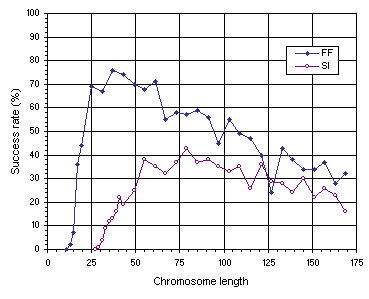Home
News
Author
Q&A
Tutorials
GEP Biblio
Contacts

Visit Gepsoft

Gene Expression Programming: Mathematical Modeling by an Artificial Intelligence

Genetic neutrality in unigenic systems

The importance of genetic neutrality in unigenic systems can be easily analyzed in GEP by increasing the gene length (Figure 7.11). After finding the most compact organization that allows the discovery of a perfect, extremely parsimonious solution to the problem at hand, any increase in gene length can lead to the evolution of perfect, less parsimonious solutions in which both neutral blocks and non-coding regions might appear.Figure 7.11. Variation of success rate with chromosome length for the function finding (FF) and sequence induction (SI) problems.

For problems exactly solved by the algorithm, the most compact organization can be found in most cases. As shown in Figure 7.11, the test function (4.1) can be compactly encoded using a head length of six (corresponding to g = 13). One such solution composed of 13 nodes is shown below:

 0123456789012 *++*//aaaaaaa

Note that, in this case, all the elements of the gene are expressed and therefore no non-coding regions exist.

The test sequence (4.9), however, requires more nodes for its correct and parsimonious expression using the chosen function set. As shown in Figure 7.11, an h = 14 (corresponding to g = 29) is the minimum head length necessary to solve this problem. The two perfect, parsimonious solutions shown below are expressed using, respectively, 25 and 23 nodes:

 01234567890123456789012345678 +*aa+*++**++/+aaaaaaaaaaaaaaa 01234567890123456789012345678 **a+a*a++/+/+/aaaaaaaaaaaaaaa

Note that the first gene has a small non-coding region composed of four elements, whereas the second has a larger non-coding region with six elements.

As Figure 7.11 emphasizes, the most compact organizations are not the most efficient. For instance, in the function finding problem, the success rate obtained for the most compact organization (g = 13) is only 2% whereas the highest success rate, obtained for a chromosome length of 37, is 76%. In the sequence induction problem, an identical behavior is observed with a success rate of only 1% for the most compact organization (g = 29) and 43% for the best chromosome length (g = 79). Therefore, a certain amount of redundancy is fundamental for evolution to occur efficiently. Indeed, in both examples, a plateau was found where the system evolves best. Note also that highly redundant systems adapt, nonetheless, considerably better than highly compact systems, showing that evolutionary systems can cope fairly well with genetic redundancy. For instance, in the function finding experiment, the most redundant system with a chromosome length of 169, has a success rate of 32%, considerably higher than the 2% obtained for the most compact organization with g = 13. The same is observed in the sequence induction experiment, where the success rate of the most redundant organization (g = 169) is 16% compared to 1% for the most compact organization (g = 29).

The structural analysis of compact organizations and less compact ones can also be useful for understanding the role of redundancy in evolution. For instance, the following perfect solutions to the function (4.1) were discovered using, respectively, head lengths of 6, 18, and 48 (only the K-expressions are shown):

 (a) 0123456789012                               *++*//aaaaaaa                           (b) 012345678901234567890123456                 +-*+/+a*/**a*/a++-aaaaaaaaa             (c) 0123456789012345678901234567890123456789...     /+aa++*+aa+*-a--+-*+*+/**a*a-*--+*/-/-/a... ...0123456789012345678901234567890123456 ...aa//-/*-aaaaaaaaaaaaaaaaaaaaaaaaaaaaa

Note that the first solution with an h = 6 is expressed using 13 nodes, and therefore the entire gene was used for its expression; the second solution with an h = 18 is expressed using 31 nodes, and therefore has a non-coding region with six elements; and the last solution with an h = 48 uses for its expression only 77 of the 97 elements of the gene and therefore has a non-coding region with 20 elements. Note also that not only the length of the non-coding region increases from the most compact to the less compact (0, 6, and 20, respectively) but also increases the number of redundant or neutral motifs. For instance, two neutral motifs using a total of 14 nodes can be counted on the medium compact solution and seven neutral motifs involving 38 nodes can be counted on the less compact one. This phenomenon is known as code bloat in genetic programming and many have argued about its evolutionary function (Angeline 1994, 1996; Nordin et al. 1995). Like all kinds of genetic redundancy, neutral motifs are most probably beneficial whenever used in good measure. This can be rigorously determined using GEP, although such an analysis would require lots of time. However, what was learned from the analysis shown in Figure 7.11 (and also from Figure 7.12 in the next section) and what is known about the evolution of proteins or technological artifacts suggest an important role for neutral regions in evolution. Their nonexistence or their excess results most probably in an inefficient evolution whereas their existence in good measure, as shown here, is beneficial.

Home | Contents | Previous | Next